﻿ 低雷诺数条件下钠冷快堆的数值模拟

# 低雷诺数条件下钠冷快堆的数值模拟Numerical Simulation of Sodium-Cooled Fast Reactor under Low Reynolds Number

Abstract: The natural cycle of sodium-cooled fast reactors is carried out under low Reynolds number conditions, so it is necessary to study the flow characteristics of sodium-cooled fast reactors under low Reynolds number conditions. The numerical simulation of the wire-wrapped rod bundle of the sodium-cooled fast reactor is carried out by using computational fluid dynamics software. The results show that the Rehme relationship is in good agreement with the simulation results. The inlet distance of the fuel assembly is less than a pitch, and the outlet effect has little effect on the friction resistance coefficient.

1. 引言

2. 几何建模及网格划分

2.1. 几何模型

2.2. 网格划分

STAR-CCM+生成的网格结构被归类为自动非结构化网格，生成的网格如图2(a)所示，从图可以看到在绕丝靠近组件壁处的网格密集；图2(b)显示的是绕丝与棒束相交之处的网格，获得这样高质量的网格是很有挑战的。以进出口压降作为选择网格的衡量指标，选择网格数在3407万与13,110万之间作一个网格无关性研究，从图3可以发现在网格数为9636万之后的压降变化很小，考虑到现有的有限计算资源，所以选择网格数为9636万的燃料组件作为研究对象来进行计算研究。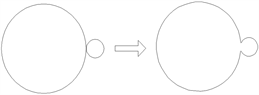(a)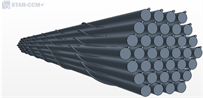(b)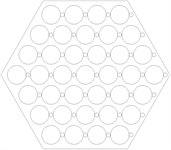(c)(d)

Figure 1. Schematic diagram of the calculation model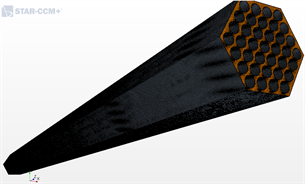(a)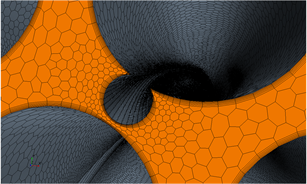(b)

Figure 2. Schematic diagram of the grid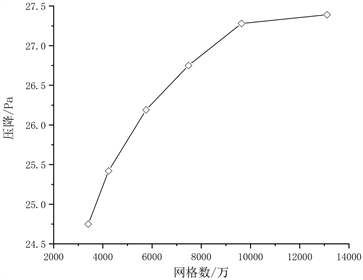Figure 3. Grid sensitivity analysis

2.3. 边界条件和计算模型设置Table 1. Physical properties of liquid sodium (T in K)

3. 计算结果及分析

$f=\frac{{C}_{fL}}{Re}\text{\hspace{0.17em}}\text{\hspace{0.17em}}\text{\hspace{0.17em}}\text{\hspace{0.17em}}Re\le R{e}_{L}$ (1)

$f=\frac{{C}_{fT}}{R{e}^{0.18}}\text{\hspace{0.17em}}\text{\hspace{0.17em}}\text{\hspace{0.17em}}\text{\hspace{0.17em}}Re\ge R{e}_{T}$ (2)

$f=\frac{{C}_{fL}}{Re}{\left(1-\psi \right)}^{1/3}+\frac{{C}_{fT}}{R{e}^{0.18}}{\psi }^{1/3}\text{\hspace{0.17em}}\text{\hspace{0.17em}}\text{\hspace{0.17em}}\text{\hspace{0.17em}}R{e}_{L}\le Re\le R{e}_{T}$ (3)

$\mathrm{log}\left(\frac{R{e}_{L}}{300}\right)=1.7\left(\frac{{P}_{t}}{{D}_{r}}-1.0\right)$

$\psi =\frac{\mathrm{log}\left(Re\right)-\left(1.7\left({P}_{t}/{D}_{r}\right)+0.78\right)}{2.52-\left({P}_{t}/{D}_{r}\right)}$

${C}_{fL}=\left(-974.6+1612.0\left(\frac{{P}_{t}}{{D}_{r}}\right)-598.5{\left(\frac{{P}_{t}}{{D}_{r}}\right)}^{2}\right)×{\left(\frac{H}{{D}_{r}+{D}_{w}}\right)}^{0.06-0.085\left({P}_{t}/{D}_{r}\right)}$

Rehme经验关系式如下：

$f=\left(\frac{64}{Re}{F}^{0.5}+\frac{0.0816}{R{e}^{0.133}}{F}^{0.9335}\right)\frac{{N}_{r}\pi \left({D}_{r}+{D}_{w}\right)}{{S}_{t}}$ (4)

$F={\left(\frac{{P}_{t}}{{D}_{r}}\right)}^{0.5}+{\left[7.6\frac{{D}_{r}+{D}_{w}}{H}{\left(\frac{{P}_{t}}{{D}_{r}}\right)}^{2}\right]}^{2.16}$

Engel经验关联式如下：

$f=\frac{110}{Re}\text{\hspace{0.17em}}\text{\hspace{0.17em}}\text{\hspace{0.17em}}\text{\hspace{0.17em}}Re<400$ (5)

$f=\frac{0.55}{R{e}^{0.25}}\text{\hspace{0.17em}}\text{\hspace{0.17em}}\text{\hspace{0.17em}}\text{\hspace{0.17em}}\text{\hspace{0.17em}}Re>5000$ (6)

$f=\frac{110}{Re}{\left(1-\psi \right)}^{0.5}+\frac{0.55}{R{e}^{0.25}}{\psi }^{0.5}\text{\hspace{0.17em}}\text{\hspace{0.17em}}\text{\hspace{0.17em}}\text{\hspace{0.17em}}\text{\hspace{0.17em}}400\le Re\le 5000$ (7)

$\psi =\frac{Re-400}{4600}$

3.1. 等温流动计算结果分析

37棒带绕丝棒束组件摩擦阻力系数计算结果示于图4。从图4中可以看出，利用层流模型计算的结果和利用k-ω湍流模型计算的结果非常的接近，两者的差距都小于0.05%以内。在Re小于600时，模拟的结果与Cheng经验关系式的结果比较接近，偏差小于13.21%；当Re在600~3000时，模拟结果与Rehme关系式的计算结果较接近，偏差小于13%；而Engel关系式计算结果整体与模拟的结果偏差较大。所以在计算类似CFR600燃料组件的带绕丝燃料棒束组件时，使用Rehme经验关系式来进行低Re条件下的阻力系数计算准确度较高。图5显示了轴向摩擦阻力系数的分布情况。从图5可以看出，摩擦阻力系数在入口区域的值要远远大于发展段的值，并且在距入口150 mm处时的摩擦阻力系数已经与充分发展段的值相差无几，说明在一个螺距之后摩擦阻力系数回复正常值，入口段效应消除；在出口处，摩擦阻力系数有明显的下降，但是变化的数值不是很大，是微小的变化，所以出口段对于摩擦阻力系数的影响是小的。同时也可以看出，在Re增大的过程中，入口段摩擦阻力系数的差距也越来越小。Figure 4. Comparison of the CFD calculation results of the friction resistance coefficient of the 37-bar-tape-wire-wound assembly with various relational expressions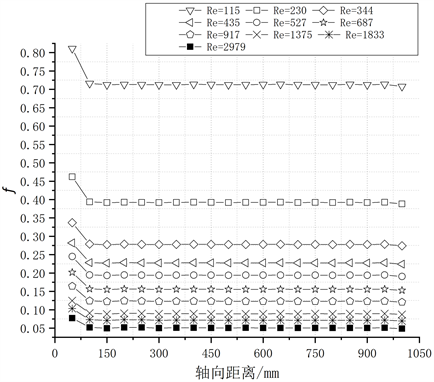Figure 5. The distribution of the axial friction coefficient of the 37-rod fuel assembly with wound wires

3.2. 棒束内部流场分析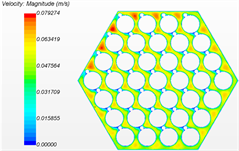(a) 25 mm处截面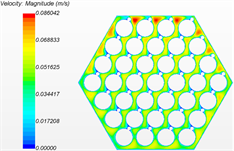(b) 50 mm处截面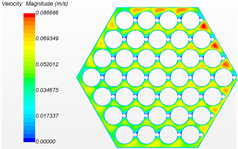(c) 75 mm处截面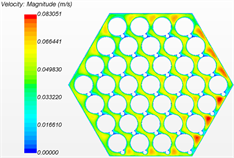(d) 100 mm处截面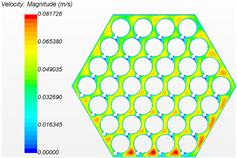(e) 125 mm处截面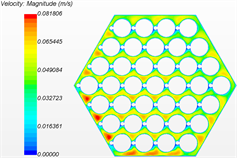(f) 150 mm处截面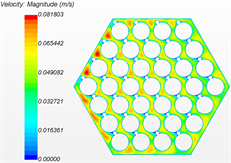(g) 175 mm处截面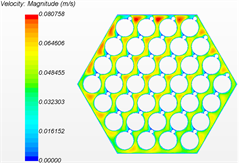(h) 200 mm处截面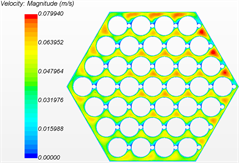(i) 225 mm处截面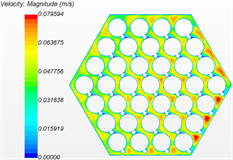(j) 250 mm处截面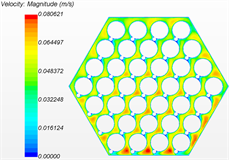(k) 275 mm处截面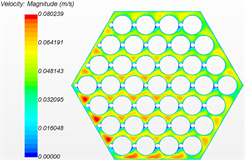(l) 300 mm处截面

Figure 6. Cloud diagram of velocity distribution of wire rod bundle

4. 结论

1) 通过不同雷诺数计算得出的摩擦阻力系数与现存的经验关系式进行对比发现，在Re小于600时，模拟的结果与Cheng经验关系式的结果比较接近，偏差小于13.21%；当Re在600~3000时，模拟结果与Rehme关系式的计算结果较接近，偏差小于13%；而Engel关系式计算结果整体与模拟的结果偏差较大。所以在计算类似CFR600燃料组件的带绕丝燃料棒束组件时，使用Rehme经验关系式来进行低Re条件下的阻力系数计算准确度较高。

2) 通过分析燃料组件轴向摩擦阻力系数分布情况发现，摩擦阻力系数在入口区域的值要远远大于发展段，在150 mm之后也就是第一个螺距之后摩擦阻力系数恢复了正常值；出口段对于摩擦阻力系数的影响较小。

3) 通过分析不同位置的速度分布云图发现，入口距离是要小于一个螺距的距离；出现最大速度的位置与绕丝和六角形壁面相对位置有关，绕丝与壁面的距离较近，则速度较大，相反速度就较小。

 Cheng, S.K. and Todreas, N.E. (1986) Hydrodynamic Models and Correlations for Bare and Wire-Wrapped Hexagonal Rod Bundles-Bundle Friction Factors, Subchannel Friction Factors and Mixing Parameters. Nuclear Engineering and Design, 92, 227-251.
https://doi.org/10.1016/0029-5493(86)90249-9

 Rehme, K. (1973) Pressure Drop Cor-relations for Fuel Element Spacers. Nuclear Technology, 17, 15-23.
https://doi.org/10.13182/NT73-A31250

 Engel, F.C., Markley, R.A. and Bishop, A.A. (2017) Laminar, Tran-sition, and Turbulent Parallel Flow Pressure Drop across Wire-Wrap-Spaced Rod Bundles. Nuclear Science and Engi-neering, 69, 290-296.
https://doi.org/10.13182/NSE79-A20618

 Hamman, K.D. and Berry, R.A. (2010) A CFD Simulation Process for Fast Reactor Fuel Assemblies. Nuclear Engineering & Design, 240, 2304-2312.
https://doi.org/10.1016/j.nucengdes.2009.11.007

 Gajapathy, R., Velusamy, K., Selvaraj, P., et al. (2007) CFD Investigation of Helical Wire-Wrapped 7-Pin Fuel Bundle and the Challenges in Modeling Full Scale 217 Pin Bundle. Nuclear Engineering and Design, 237, 2332-2342.
https://doi.org/10.1016/j.nucengdes.2007.05.003

 刘洋, 喻宏, 周志伟. 钠冷快堆燃料组件热工水力特性数值模拟与分析[J]. 原子能科学技术, 2014(10): 1790-1796.

 李淞, 杨红义, 周志伟, 等. 快堆组件稠密棒束数值模拟[J]. 原子能科学技术, 2018, 52(4): 41-45.

 Nina, Y., Dalin, Z., Jing, C., et al. (2018) The Development and Validation of the inteRwrapper Flow Model in Sodium-Cooled Fast Reactors. Progress in Nuclear Energy, 108, 54-65.
https://doi.org/10.1016/j.pnucene.2018.05.007

 刘亮. 从“十三五”规划看电力转型发展[J]. 大众用电, 2019, 34(4): 3-4.

 赵成昆. 中国核电建设进入全新时代[J]. 中国电力企业管理, 2019(4): 37-39.

 曹殿鹏, 邹树梁, 唐德文, 肖魏魏, 于洁. 三种核燃料循环方案的综合效益比较[J]. 核科学与工程, 2019, 39(5): 809-820.

Top